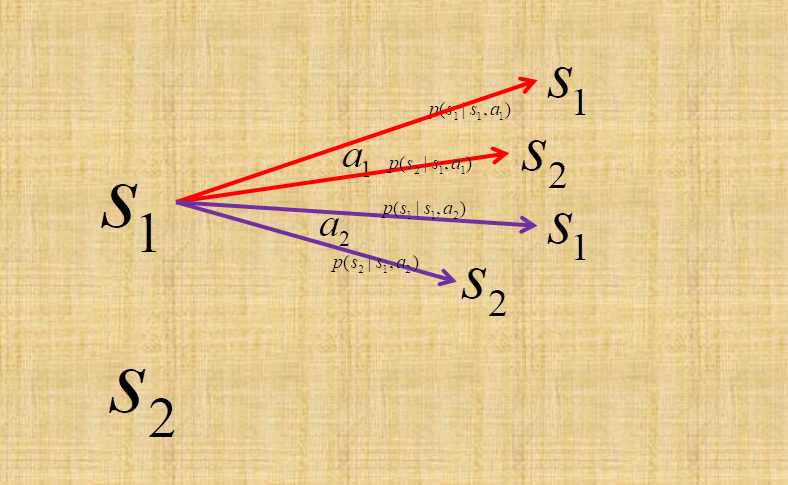# 强化学习（强化主干知识，看这篇就够了）

## 强化学习

### 文章目录

#### 核心内容

###### 元素简介R : S × A × S ↦ R R : S \times A \times S \mapsto \mathbb{R} 指定了奖赏。这个奖赏不仅跟起始状态和动作有关，也和动作执行后达到的状态有关。

###### 优化目标：状态值函数

{ V T π ( s ) = E π [ 1 T ∑ t = 1 T r t ∣ s 0 = s ] V γ π ( s ) = E π [ ∑ t = 0 + ∞ γ t r t + 1 ∣ s 0 = s ] \left\{\begin{array}{l}{V_{T}^{\pi}(s)=\mathbb{E}_{\pi}\left[\frac{1}{T} \sum_{t=1}^{T} r_{t} | s_{0}=s\right]} \\ {V_{\gamma}^{\pi}(s)=\mathbb{E}_{\pi}\left[\sum_{t=0}^{+\infty} \gamma^{t} r_{t+1} | s_{0}=s\right]}\end{array}\right.

V T π ( s ) = E π [ 1 T ∑ t = 1 T r t ∣ s 0 = s ] = E π [ 1 T r 1 + T − 1 T 1 T − 1 ∑ t = 2 T r t ∣ s 0 = s ] = ∑ a ∈ A π ( s , a ) ∑ s ′ ∈ S P ( s ′ ∣ s , a ) ( 1 T R ( s ′ ∣ s , a ) + T − 1 T E π [ 1 T − 1 ∑ t = 1 T − 1 r t ∣ s 0 = s ′ ] ) = ∑ a ∈ A π ( s , a ) ∑ s ′ ∈ S P ( s ′ ∣ s , a ) ( 1 T R ( s ′ ∣ s , a ) + T − 1 T V T − 1 π ( s ′ ) ) \begin{aligned} V_{T}^{\pi}(s) &amp;=\mathbb{E}_{\pi}\left[\frac{1}{T} \sum_{t=1}^{T} r_{t} | s_{0}=s\right] \\ &amp;=\mathbb{E}_{\pi}\left[\frac{1}{T} r_{1}+\frac{T-1}{T} \frac{1}{T-1} \sum_{t=2}^{T} r_{t} | s_{0}=s\right] \\ &amp;=\sum_{a \in A} \pi(s, a) \sum_{s^{\prime} \in S} P(s^{\prime}|s,a)\left(\frac{1}{T} R(s&#x27;|s,a)+\frac{T-1}{T} \mathbb{E}_{\pi}\left[\frac{1}{T-1} \sum_{t=1}^{T-1} r_{t} | s_{0}=s^{\prime}\right]\right) \\ &amp;=\sum_{a \in A} \pi(s, a) \sum_{s^{\prime} \in S} P(s^{\prime}|s,a)\left(\frac{1}{T} R(s&#x27;|s,a)+\frac{T-1}{T} V_{T-1}^{\pi}\left(s^{\prime}\right)\right) \end{aligned}

V γ π ( s ) = ∑ a ∈ A π ( s , a ) ∑ s ′ ∈ S P ( s ′ ∣ s , a ) ( R ( s ′ ∣ s , a ) + γ V γ π ( s ′ ) ) V_{\gamma}^{\pi}(s)=\sum_{a \in A} \pi(s, a) \sum_{s^{\prime} \in S} P(s^{\prime}|s,a)\left(R(s&#x27;|s,a)+\gamma V_{\gamma}^{\pi}\left(s^{\prime}\right)\right)

###### 状态-动作值函数

{ Q T π ( s , a ) = ∑ s ′ ∈ S P ( s ′ ∣ s , a ) ( 1 T R ( s ′ ∣ s , a ) + T − 1 T V T − 1 π ( s ′ ) ) Q γ π ( s , a ) = ∑ s ′ ∈ S P ( s ′ ∣ s , a ) ( R ( s ′ ∣ s , a ) + γ V γ π ( s ′ ) ) \left\{\begin{array}{l}{Q_{T}^{\pi}(s, a)=\sum_{s^{\prime} \in S} P(s^{\prime}|s,a)\left(\frac{1}{T} R(s&#x27;|s,a)+\frac{T-1}{T} V_{T-1}^{\pi}\left(s^{\prime}\right)\right)} \\ {Q_{\gamma}^{\pi}(s, a)=\sum_{s^{\prime} \in S} P(s^{\prime}|s,a)\left(R(s&#x27;|s,a)+\gamma V_{\gamma}^{\pi}\left(s^{\prime}\right)\right)}\end{array}\right.

#### 策略迭代和值迭代

###### 值迭代

V ∗ ( s ) = max ⁡ a ∈ A Q ∗ ( s , a ) V^{*}(s)=\max _{a \in A} Q^{{*}}(s, a)

{ V T ∗ ( s ) = max ⁡ a ∈ A ∑ s ′ ∈ S P ( s ′ ∣ s , a ) ( 1 T R ( s ′ ∣ s , a ) + T − 1 T V T − 1 ∗ ( s ′ ) ) V γ ∗ ( s ) = max ⁡ a ∈ A ∑ s ′ ∈ S P ( s ′ ∣ s , a ) ( R ( s ′ ∣ s , a ) + γ V γ ∗ ( s ′ ) ) \left\{\begin{array}{l}{V_{T}^{*}(s)=\max _{a \in A} \sum_{s^{\prime} \in S} P(s^{\prime}|s,a)\left(\frac{1}{T} R(s&#x27;|s,a)+\frac{T-1}{T} V_{T-1}^{*}\left(s^{\prime}\right)\right)} \\ {V_{\gamma}^{*}(s)=\max _{a \in A} \sum_{s^{\prime} \in S} P(s^{\prime}|s,a)\left(R(s&#x27;|s,a)+\gamma V_{\gamma}^{*}\left(s^{\prime}\right)\right)}\end{array}\right.

{ Q T ∗ ( s , a ) = ∑ s ′ ∈ S P ( s ′ ∣ s , a ) ( 1 T R ( s ′ ∣ s , a ) + T − 1 T max ⁡ a ′ ∈ A Q T − 1 ∗ ( s ′ , a ′ ) ) Q γ ∗ ( s , a ) = ∑ s ′ ∈ S P ( s ′ ∣ s , a ) ( R ( s ′ ∣ s , a ) + γ max ⁡ a ′ ∈ A Q γ ∗ ( s ′ , a ′ ) ) \left\{\begin{array}{l}{Q_{T}^{*}(s, a)=\sum_{s^{\prime} \in S} P(s^{\prime}|s,a)\left(\frac{1}{T} R(s&#x27;|s,a)+\frac{T-1}{T} \max _{a^{\prime} \in A} Q_{T-1}^{*}\left(s^{\prime}, a^{\prime}\right)\right)} \\ {Q_{\gamma}^{*}(s, a)=\sum_{s^{\prime} \in S} P(s^{\prime}|s,a)\left(R(s&#x27;|s,a)+\gamma \max _{a^{\prime} \in A} Q_{\gamma}^{*}\left(s^{\prime}, a^{\prime}\right)\right)}\end{array}\right.

#### Q学习、SARSA、时序差分学习

###### Q学习

Q学习算法，需要了解Q表。Q-table 其实就是把状态和动作一个作为行标，一个作为列标搞成表格。我们要求状态-动作值函数（ Q Q 函数）其实也就是要想办法求出最优动作下的Q表。

###### SARSA和时序差分学习

Sarsa是Q学习算法的改进。Sarsa和Q以及TD本质上都是一脉同根的。

#### 一些学习资料

10-257247
05-22119302-288907
08-27270
01-121561
03-105382
04-132059
05-20518
05-111627
03-29222
03-07828
07-11522
05-15336